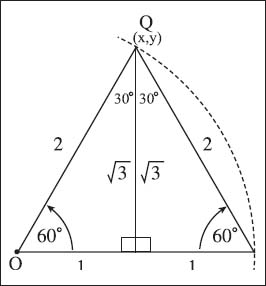NextPrevious

# What are some identities in trigonometry?

There are many fundamental identities—an equation that is true regardless of what values are substituted for any of the variables—based on trigonometric functions. Because there are relationships between the trig functions, identities can be used to rewrite equations, allowing the user to simplify or get more information out of an equation. The identities include reciprocal identities, ratio identities, periodicity identities, Pythagorean identities, odd-even identities, sum-difference identities, double angle identities, half angle identities, and several more. The following lists a few such identities:

Pythagorean Identities

Reciprocal Identities

Ratio (or Quotient) Identitiesin this example, to find the trig functions of an angle of 60 degrees, draw a triangle with point O at the center of a circle with radius = 2; use the Pythagorean theorem to determine the length of the line dropping down at a right angle from point Q; and then calculate the trig functions using these measurements.

Close

This is a web preview of the "The Handy Math Answer Book" app. Many features only work on your mobile device. If you like what you see, we hope you will consider buying. Get the App